Home > ACC7 > Chapter cc38 > Lesson cc38.3.1 > Problem8-132

8-132.
1. A candy store’s specialty is taffy. Customers can fill a bag with taffy, and the price is based on how much the candy weighs. The store charges $2 for 10 ounces (oz) of taffy. 8-132 HW eTool (Desmos). Homework Help ✎ 1. Copy the table below and fill in the missing values. Add three more entries.  Amount of Taffy (oz) 2 5 10 12 15 20 Price ($) $2$4
2. Graph the values in the table. Let x represent the number of ounces and y represent the price in dollars.

3. Is this situation proportional? Explain your reasoning.

4. What is the slope of the line you graphed? What information does the slope tell you?

5. Write the equation that represents the candy store’s pricing.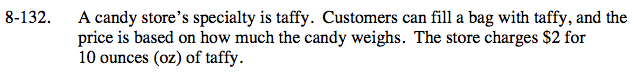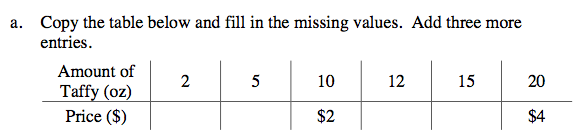Here are two of the answers: (5, $1) and (12,$2.40). Fill in the rest of the table, and add three more entries.
You may find using proportions to be helpful in finding more answers.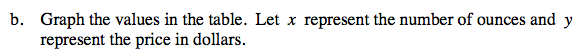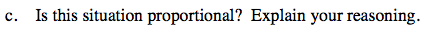Does the data make a straight line through (0,0)?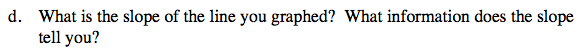$\text{Find the slope using }\frac{\text{rise}}{\text{run}}.$

Find the price for 1 ounce of taffy. What do you notice?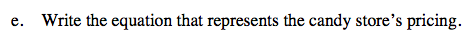Use the equation y = mx+b, where:
m = slope
b = y-intercept

Complete the table in the etool below to graph the values.
Click the link at right for the full version of the eTool: CC3 8-132 HW eTool